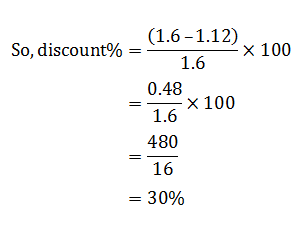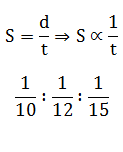# SSC Quantitative Aptitude Questions (Day-54)

Dear Aspirants, you can find the Quantitative Aptitude questions with detailed explanations for the SSC exams. Nowadays the competitive level of the exam has been increasing consistently. Due to the great demand for the government job, the level of the toughness reached greater. Candidates have to enhance the preparation process in order to drive in the right path. It doesn’t need to clear the prescribed cutoff. You must have to score good marks more than the cut off marks to get into the final provisional list. Here we have updating the Quantitative Aptitude questions with detailed explanations on a daily basis. You can practice with us and measure your level of preparation. According to that you can sculpt yourself in a proper way. SSC aspirants kindly make use of it and grab your success in your career.

Start Quiz

1) If N = (√7 – √5)/(√7 + √5), then what is the value of 1/N?

(a) 6 – √35

(b) 6 + √35

(c) 7 + √35

(d) 7 – √35

2) The sum of three consecutive even numbers is always divisible by ____.

(a) 12

(b) 6

(c) 18

(d) 24

3) How many positive factors of 24 are there?

(a) 3

(b) 4

(c) 6

(d) 8

4) 4/5 part of a tank is filled with oil. After taking out 42 litres of oil the tank is 3/4 part full. What is the capacity (in litres) of the tank?

(a) 420

(b) 630

(c) 840

(d) 1680

5) What is the largest fourdigit number which is a perfect square?

(a) 9704

(b) 9801

(c) 9901

(d) 9999

6) Two inlet pipes can fill a cistern in 20 and 24 hours respectively and an outlet pipe can empty 160 gallons of water per hour. All the three pipes workingtogether can fill the empty cistern in 40 hours. What is the capacity (in gallons) of the tank?

(a) 1200

(b) 2400

(c) 3600

(d) 1800

7) P alone can complete the work in 5 days, Q alone can do same work in 6 days and R alone can do the same work in 12 days. They jointly complete thework and earn Rs 5400. What is the share of R?

(a) 1000

(b) 1200

(c) 1500

(d) 1800

8) After giving two successive discounts of 20% and 25% a cycle is sold for Rs 4200. What is the marked price (in Rs) of the cycle?

(a) 7200

(b) 7000

(c) 6500

(d) 6200

9) The marked price of an article is 60% more than its cost price. What should be the discount (in %) offered by the shopkeeper so that he earns a profitof 12%?

(a) 12

(b) 25

(c) 30

(d) 60

10) The ratio of the speed of P, Q and R is 10 : 12 : 15 respectively. What is the ratio of the time taken by P, Q and R respectively to cover the samedistance?

(a) 10 : 12 : 15

(b) 15 : 12 : 10

(c) 6 : 5 : 4

(d) 4 : 5 : 6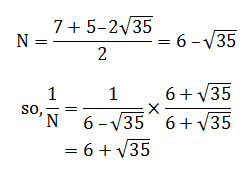Smallest consecutive three even numbers.

2 + 4 + 6 = 12.

Cleary, 12 is divisible either by 6 or 12.

Now, next three consecutive nos 4 + 6 + 8 = 18

Which is divisible only by 6.

24 = 2 × 2 × 2 × 3 = 2³ × 3¹

So factors = (3 + 1) × (1 + 1)

= 4 × 2

= 8

Let capacity be x

So,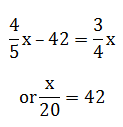or x = 840ℓ

We know that 100² = 10000.

So, largest four digit perfect square = 99²

= (100 – 1)²

= 9801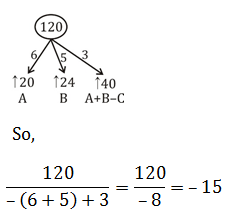∴ C empties tank in 15 hrs

So, if 160 gallons = 1 hr

So 15 hrs = 2400 gallons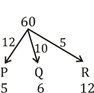So, ratio of efficiency 12:10:5.

So,

Share of R = 5/27 × 5400 =1000

Net discount = 20+25 – (20 × 25)/100

= 45 –5

= 40%

∴ 0.6x = 4200

x = 7000

MP = 1.6 CP

SP = 1.12 CP